# DATEVALUE Function

Converts imported dates from text to proper date format

## What is the DATEVALUE Function?

The DATEVALUE Function is categorized under Excel Date/Time functions. When doing financial analysis, we often need to import files from another source, which can be in PDF, text, or csv file format. The DATEVALUE function helps convert dates imported from external sources that are in text format to a proper date format recognizable by Excel for further analysis.

### Formula

=DATEVALUE(date_text)

The DATEVALUE function includes the following arguments:

Date_text – This is a required argument. It is the text that represents a date in an Excel date format, or a reference to a cell that contains text that represents a date in an Excel date format. For example, “1/30/2017” or “30-Jan-2017” are text strings within quotation marks that represent dates.

### How to use the DATEVALUE Function in Excel?

To understand the uses of this function, let’s consider a few examples:

#### Example 1

Suppose we have been given following dates in text format. We shall use this function to convert the different text representations of dates given to us.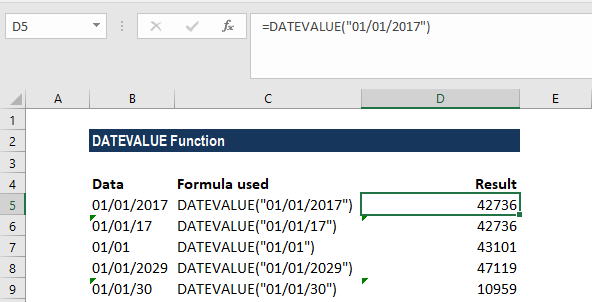The DATEVALUE function in Excel, when interpreting the supplied date_text argument, follows simple rules to interpret the year. The rules are:

• When we provide just a date and month, then the returned date will return the current year. This function will take the current year from the computer’s system clock.
• For this function, the default settings for the year are the one- and two-digit years interpreted as follows:
• The numbers 0 through to 29 are interpreted as the years 2000 to 2029;
• The numbers 30 through to 99 are interpreted as the years 1930 to 1999.

As seen above, when we provide 01/01/30, this function interpreted it as 1930. The results, as shown above, are returned as numbers due to the “General” formatting type. So, the results displayed above show the underlying numeric value for the resulting dates. We need to change the formatting to DATE to get dates in the result column.  For this, we can select the cell or range of cells and, on the Home tab, click on number format and select Date instead of Number, as shown below: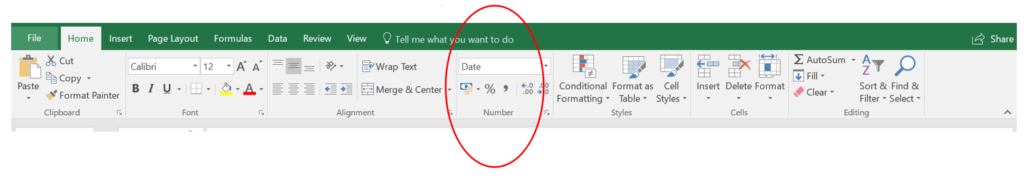The other way to do it is to select a cell or a range of cells with the numbers that we want to convert to dates and then press Ctrl+1 to open the Format Cells dialog. On the Number tab, choose Date and select the desired date format under Type and click OK.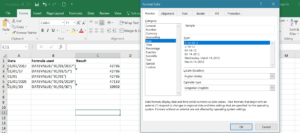The result we get is as follows: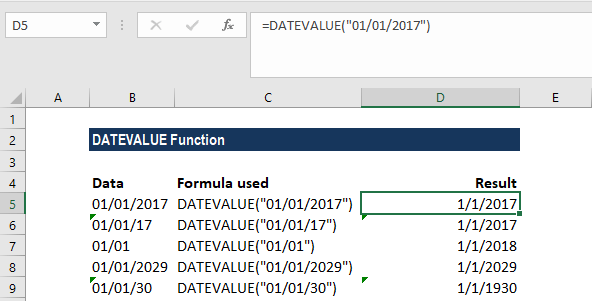#### Example 2

Taking the same dates in the example above, we added the time factor to them as shown below: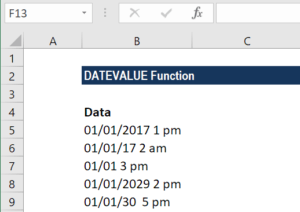Let’s see how this function behaves in such a scenario. The formula used is DATEVALUE(A1). The results are shown below: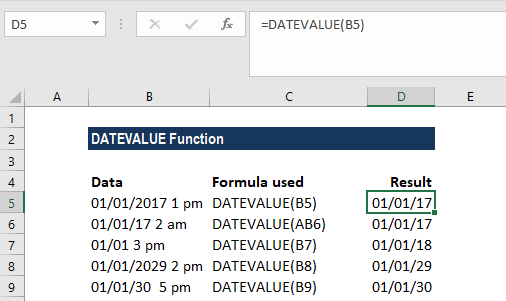As we can see, this function ignores the time information in text strings.  If we wish to convert text values containing both dates and times, we need to use the VALUE function.

#### Example 3

Let’s assume that while importing the data from a PDF, we obtained the dates in the following format: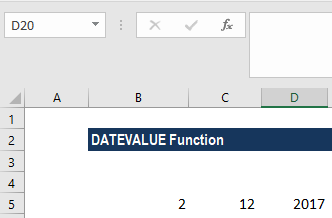Using the DATEVALUE function, we can combine the above data to get a single date in a cell. The formula to be used is =DATEVALUE(A2 & “/” & B2 & “/” & C2) as shown below: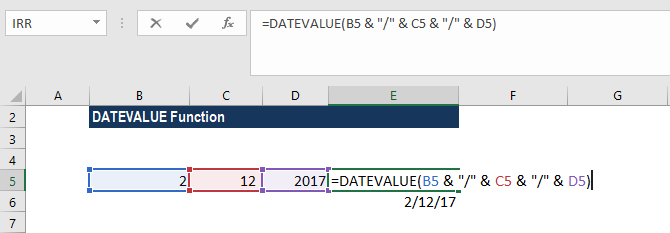We get the result below: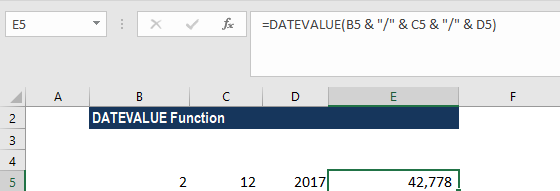We can format the result in date format to get the result as: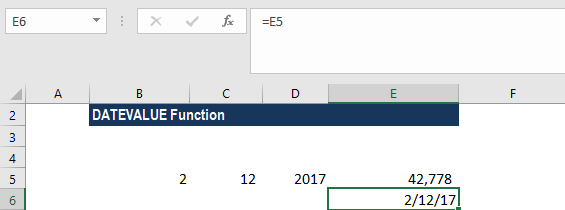### Notes about the DATEVALUE Function

1. #NUM! error – Occurs when the start_date is greater than the end_date.
2. #VALUE! Error – Occurs when the value of the date_text argument falls outside of the range January 1, 1900 and December 31, 9999.

Thanks for reading CFI’s guide to important Excel functions! By taking the time to learn and master these functions, you’ll significantly speed up your financial analysis. To learn more, check out these additional CFI resources:

• Excel Functions for Finance